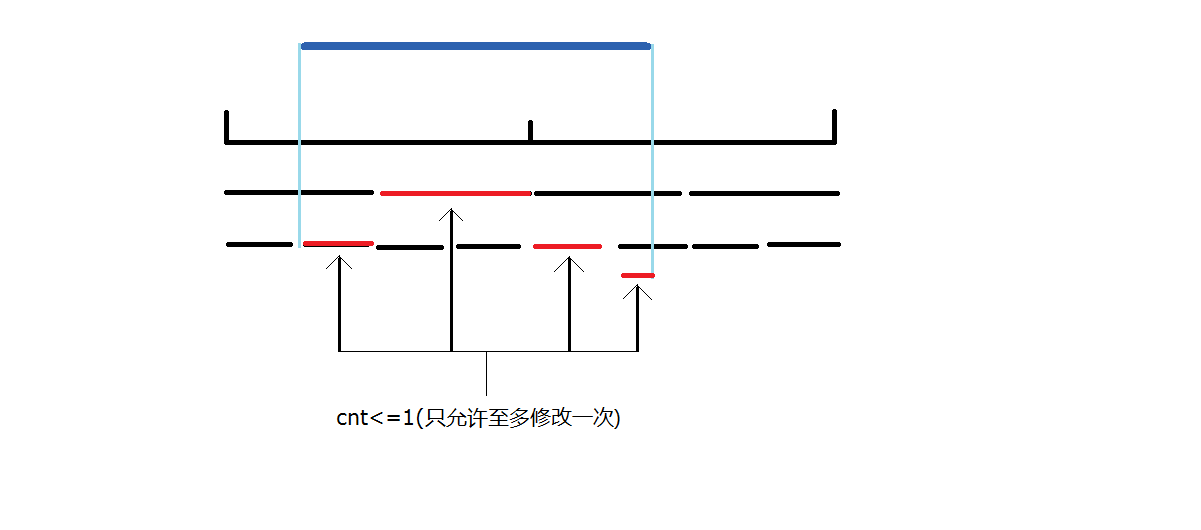题目

题目

Bash likes playing with arrays. He has an array $a_1$, $a_2$, … an of n integers. He likes to guess the greatest common divisor (gcd) of different segments of the array. Of course, sometimes the guess is not correct. However, Bash will be satisfied if his guess is almost correct.

Suppose he guesses that the gcd of the elements in the range $[l, r]$ of a is x. He considers the guess to be almost correct if he can change at most one element in the segment such that the gcd of the segment is x after making the change. Note that when he guesses, he doesn’t actually change the array — he just wonders if the gcd of the segment can be made x. Apart from this, he also sometimes makes changes to the array itself.

Since he can’t figure it out himself, Bash wants you to tell him which of his guesses are almost correct. Formally, you have to process q queries of one of the following forms:

$1 l r x$ — Bash guesses that the gcd of the range $[l, r]$ is x. Report if this guess is almost correct.
$2 i y$ — Bash sets ai to y.
Note: The array is 1-indexed.

Input
The first line contains an integer n $(1 ≤ n ≤ 5·10^5)$ — the size of the array.

The second line contains n integers $a_1, a_2, ..., a_n$ $(1 ≤ a_i ≤ 10^9)$ — the elements of the array.

The third line contains an integer q $(1 ≤ q ≤ 4·10^5)$ — the number of queries.

The next q lines describe the queries and may have one of the following forms:

$1 l r x \quad(1 ≤ l ≤ r ≤ n, 1 ≤ x ≤ 10^9).$
$2 i y \quad \quad(1 ≤ i ≤ n, 1 ≤ y ≤ 10^9).$
Guaranteed, that there is at least one query of first type.

Output
For each query of first type, output “YES” (without quotes) if Bash’s guess is almost correct and “NO” (without quotes) otherwise.

Examples
Input

3
2 6 3
4
1 1 2 2
1 1 3 3
2 1 9
1 1 3 2


Output

YES
YES
NO


Input

5
1 2 3 4 5
6
1 1 4 2
2 3 6
1 1 4 2
1 1 5 2
2 5 10
1 1 5 2


Output

NO
YES
NO
YES


Note
In the first sample, the array initially is {2, 6, 3}.

For query 1, the first two numbers already have their gcd as 2.

For query 2, we can achieve a gcd of 3 by changing the first element of the array to 3. Note that the changes made during queries of type 1 are temporary and do not get reflected in the array.

After query 3, the array is now {9, 6, 3}.

For query 4, no matter which element you change, you cannot get the gcd of the range to be 2.

题目大意

1 l r x:查询区间$[l,r]$是否能通过不改变或只临时改变其中一个元素的值使得
$gcd(a[i])=x(i∈[l,r])$输出"YES"或“NO”
2 i y:将a[i]值改为y

思路

$Gcd[l,r]=gcd(Gcd[l,mid],Gcd[mid+1,r])$代码

常规版

//343ms 23500kB
#include<set>
#include<map>
#include<ctime>
#include<queue>
#include<cmath>
#include<cstdio>
#include<vector>
#include<climits>
#include<cstring>
#include<iostream>
#include<algorithm>
#define LL long long
using namespace std;
int f=1,x=0;char s=getchar();
while(s<'0'||s>'9'){if(s=='-')f=-1;s=getchar();}
while(s>='0'&&s<='9'){x=x*10+s-'0';s=getchar();}
return x*f;
}
#define lch i<<1
#define rch i<<1|1
#define MAXN 500000
#define INF 0x3f3f3f3f
#define Mod int(1e9+7)
struct Tree{
int l,r,gcd;
Tree(){}
Tree(int L,int R,int G){l=L,r=R,gcd=G;}
}tree[MAXN<<2];
inline int gcd(int a,int b){return !b?a:gcd(b,a%b);}
void Build(int i,int l,int r){
tree[i].l=l,tree[i].r=r;
if(l==r){
return ;
}
int mid=(l+r)>>1;
Build(lch,l,mid);
Build(rch,mid+1,r);
tree[i].gcd=gcd(tree[lch].gcd,tree[rch].gcd);
return ;
}
void Update(int i,int p,int x){
if(tree[i].l==p&&tree[i].r==p){
tree[i].gcd=x;
return ;
}
int mid=(tree[i].l+tree[i].r)>>1;
if(p<=mid) Update(lch,p,x);
else Update(rch,p,x);
tree[i].gcd=gcd(tree[lch].gcd,tree[rch].gcd);
return ;
}
int cnt;
void Query(int i,int L,int R,int x){
if(cnt>1) return ;
if(L<=tree[i].l&&tree[i].r<=R){
if(tree[i].gcd%x==0)
return ;
else if(tree[i].l==tree[i].r){
cnt++;
return ;
}
int lg=tree[lch].gcd,rg=tree[rch].gcd;
if(lg%x&&rg%x){
cnt=2;
return ;
}
if(lg%x)
Query(lch,L,R,x);
if(rg%x)
Query(rch,L,R,x);
return ;
}
int mid=(tree[i].l+tree[i].r)>>1;
if(L<=mid) Query(lch,L,R,x);
if(mid+1<=R) Query(rch,L,R,x);
return ;
}
int main(){
Build(1,1,n);
for(int i=1;i<=q;i++){
}
return 0;
}


懒人加速版

//296 ms7800 KB
#include<set>
#include<map>
#include<ctime>
#include<queue>
#include<cmath>
#include<cstdio>
#include<vector>
#include<climits>
#include<cstring>
#include<iostream>
#include<algorithm>
#define LL long long
using namespace std;
int f=1,x=0;char s=getchar();
while(s<'0'||s>'9'){if(s=='-')f=-1;s=getchar();}
while(s>='0'&&s<='9'){x=x*10+s-'0';s=getchar();}
return x*f;
}
#define lch i<<1
#define rch i<<1|1
#define MAXN 500000
#define INF 0x3f3f3f3f
#define Mod int(1e9+7)
int Gcd[MAXN<<2];
inline int gcd(int a,int b){return !b?a:gcd(b,a%b);}
void Build(int i,int l,int r){
if(l==r){
return ;
}
int mid=(l+r)>>1;
Build(lch,l,mid);
Build(rch,mid+1,r);
Gcd[i]=gcd(Gcd[lch],Gcd[rch]);//向上更新
return ;
}
void Update(int i,int l,int r,int p,int x){
if(l==p&&r==p){
Gcd[i]=x;
return ;
}
int mid=(l+r)>>1;
if(p<=mid) Update(lch,l,mid,p,x);
else Update(rch,mid+1,r,p,x);
Gcd[i]=gcd(Gcd[lch],Gcd[rch]);
return ;
}
int cnt;
void Query(int i,int l,int r,int L,int R,int x){
if(cnt>1) return ;//不符合直接返回
int mid=(l+r)>>1;
if(L<=l&&r<=R){//该区间为查询区间子区间
if(Gcd[i]%x==0)//子区间符合条件
return ;
else if(l==r){//为叶节点
cnt++;
return ;
}//子区间Gcd%x!=0,cnt必定会改变
int lg=Gcd[lch],rg=Gcd[rch];
if((lg%x&&rg%x)||cnt){//两区间不符条件或cnt已有值
cnt=2;
return ;
}//此时只有一个子区间不满足条件
if(lg%x) Query(lch,l,mid,L,R,x);//递归处理
if(rg%x) Query(rch,mid+1,r,L,R,x);
return ;
}
if(L<=mid) Query(lch,l,mid,L,R,x);//找子区间
if(mid+1<=R) Query(rch,mid+1,r,L,R,x);
return ;
}
int main(){